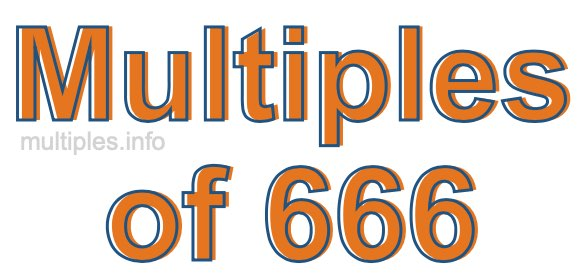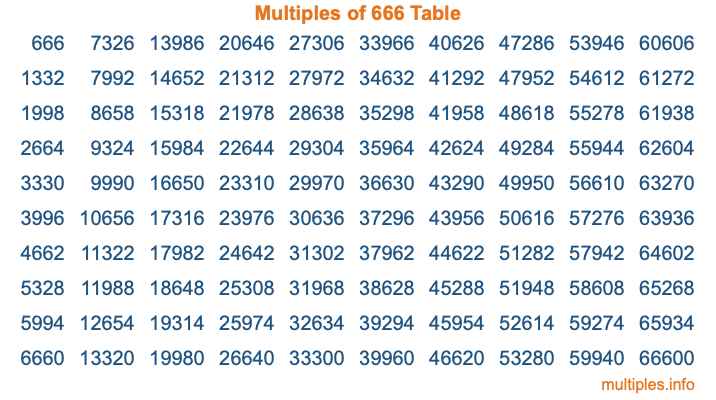Multiples of 666Welcome to the Multiples of 666 page. Here we will first teach you everything you will ever need to know about the multiples of 666, and then give you a study guide summary of everything we taught you to make sure you remember it all. Use this page to look up facts and learn information about the multiples of 666. This page will make you a multiples of six hundred sixty-six expert!

Definition of Multiples of 666
Multiples of 666 are all the numbers that when divided by 666 equal an integer. Each of the multiples of 666 are called a multiple. A multiple of 666 is created by multiplying 666 by an integer.

Therefore, to create a list of multiples of 666, you start with 1 multiplied by 666, then 2 multiplied by 666, then 3 multiplied by 666, and so on for as long as you want. Thus, the list of the first five multiples of 666 is 666, 1332, 1998, 2664, and 3330. To see a larger list of multiples of 666, see the printable image of Multiples of 666 further down on this page. We also have a category where you can choose any nth multiple of 666.

Multiples of 666 Checker
The Multiples of 666 Checker below checks to see if any number of your choice is a multiple of 666. In other words, it checks to see if there is any number (integer) that when multiplied by 666 will equal your number. To do that, we divide your number by 666. If the the quotient is an integer, then your number is a multiple of 666.

Is  a multiple of 666?

Least Common Multiple of 666 and ...
A Least Common Multiple (LCM) is the lowest multiple that two or more numbers have in common. This is also called the smallest common multiple or lowest common multiple and is useful to know when you are adding our subtracting fractions. Enter one or more numbers below (666 is already entered) to find the LCM.

Check out our LCM Calculator if you need more details about the Least Common Multiple or if you need the LCM for different numbers for adding and subtraction fractions.

nth Multiple of 666
As we stated above, 666 is the first multiple of 666, 1332 is the second multiple of 666, 1998 is the third multiple of 666, and so on. Enter a number below to find the nth multiple of 666.

th multiple of 666

Multiples of 666 vs Factors of 666
666 is a multiple of 666 and a factor of 666, but that is where the similarities end. All postive multiples of 666 are 666 or greater than 666. All positive factors of 666 are 666 or less than 666.

Below is the beginning list of multiples of 666 and the factors of 666 so you can compare:

Multiples of 666: 666, 1332, 1998, 2664, 3330, etc.

Factors of 666: 1, 2, 3, 6, 9, 18, 37, 74, 111, 222, 333, 666

As you can see, the multiples of 666 are all the numbers that you can divide by 666 to get a whole number. The factors of 666, on the other hand, are all the whole numbers that you can multiply by another whole number to get 666.

It's also interesting to note that if a number (x) is a factor of 666, then 666 will also be a multiple of that number (x).

Multiples of 666 vs Divisors of 666
The divisors of 666 are all the integers that 666 can be divided by evenly. Below is a list of the divisors of 666.

Divisors of 666: 1, 2, 3, 6, 9, 18, 37, 74, 111, 222, 333, 666

The interesting thing to note here is that if you take any multiple of 666 and divide it by a divisor of 666, you will see that the quotient is an integer.

Multiples of 666 Table
Below is an image of the first 100 multiples of 666 in a table. The table is in chronological order, column by column. The first column has the first ten multiples of 666, the second column has the next ten multiples of 666, and so on.The Multiples of 666 Table is also referred to as the 666 Times Table or Times Table of 666. You are welcome to print out our table for your studies.

Negative Multiples of 666
Although not often discussed or needed in math, it is worth mentioning that you can make a list of negative multiples of 666 by multiplying 666 by -1, then by -2, then by -3, and so on, to get the following list of negative multiples of 666:

-666, -1332, -1998, -2664, -3330, etc.

Multiples of 666 Summary
Below is a summary of important Multiples of 666 facts that we have discussed on this page. To retain the knowledge on this page, we recommend that you read through the summary and explain to yourself or a study partner why they hold true.

There are an infinite number of multiples of 666.

A multiple of 666 divided by 666 will equal a whole number.

666 divided by a factor of 666 equals a divisor of 666.

The nth multiple of 666 is n times 666.

The largest factor of 666 is equal to the first positive multiple of 666.

666 is a multiple of every factor of 666.

666 is a multiple of 666.

A multiple of 666 divided by a divisor of 666 equals an integer.

666 divided by a divisor of 666 equals a factor of 666.

Any integer times 666 will equal a multiple of 666.

Multiples of a Number
Here you can get the multiples of another number, all with the same attention to detail as we did for multiples of 666 on this page.

Multiples of
Multiples of 667
Did you find our page about multiples of six hundred sixty-six educational? Do you want more knowledge? Check out the multiples of the next number on our list!